# Amsco Geometry Chapter 13: Geometry of a Circle Chapter Exam

Exam Instructions:

Choose your answers to the questions and click 'Next' to see the next set of questions. You can skip questions if you would like and come back to them later with the yellow "Go To First Skipped Question" button. When you have completed the practice exam, a green submit button will appear. Click it to see your results. Good luck!

### Page 1

#### Question 2 2. In the picture below, if arc BD is 168 degrees and arc BC is 70 degrees, what is the measure of angle BAD?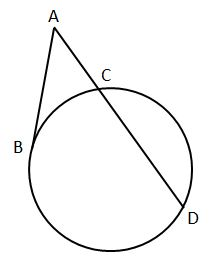#### Question 5 5. Find the center and radius of the following equation: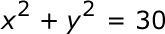### Page 2

#### Question 6 6. If angle AOB is 40 degrees, what is the measure of angle ACB?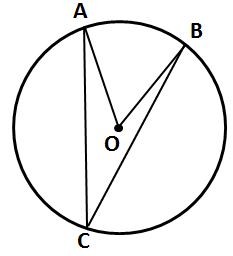#### Question 8 8. What is the area of the shaded area, rounded to the nearest whole number?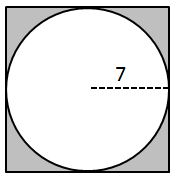### Page 3

#### Question 11 11. If angle ACB is 47 degrees, what is the measure of angle AOB?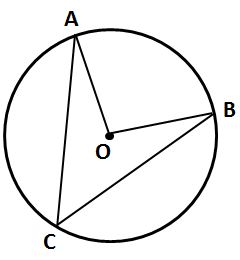#### Question 13 13. If arc AB is 72 degrees, what is the measure of angle ACB?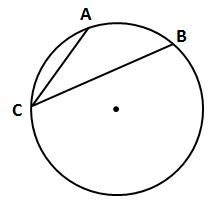#### Question 14 14. In the picture below, if arc AB is 82 degrees, what is the measure of angle BAC?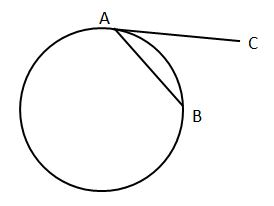### Page 4

#### Question 20 20. What is the line, as shown in the picture, called?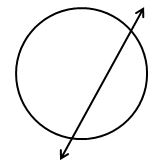### Page 5

#### Question 21 21. In the picture below, if arc CE is 172 degrees and arc BD is 80 degrees, what is the measure of angle CAE?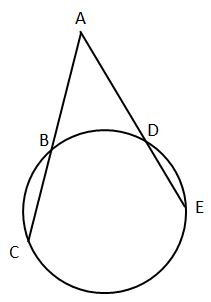#### Question 23 23. In the picture below, if arc AC is 146 degrees and arc BD is 32 degrees, what is the measure of angle AEC?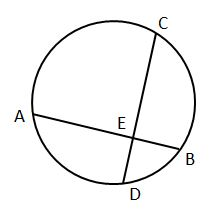### Page 6

#### Question 26 26. In the pictured circle, the measure of angle ACB is 22 degrees and the measure of angle BXD is 120 degrees. What is the measure of angle CAD?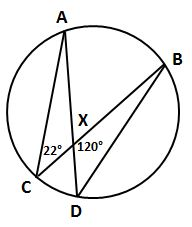#### Question 28 28. If angle ACB is 34 degrees, what is the measure of angle ADB?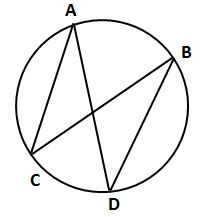#### Question 29 29. If the measure of central angle AOB is 62 degrees, what is the measure of inscribed angle ACB?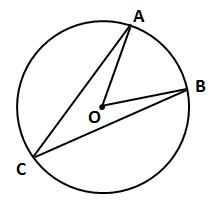#### Amsco Geometry Chapter 13: Geometry of a Circle Chapter Exam Instructions

Choose your answers to the questions and click 'Next' to see the next set of questions. You can skip questions if you would like and come back to them later with the yellow "Go To First Skipped Question" button. When you have completed the practice exam, a green submit button will appear. Click it to see your results. Good luck!

Support